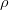## Notes about valves and flow rates

Comparing valves for compressed gases can be difficult for several reasons. Even if you are familiar with how valve performance is specified, you can easily make mistakes. Valve performance is specified in several different sloppy and confusing ways, almost always in non-metric units. The worst part is that valve performance can be specified in obvious ways, but people working in the industry don’t do that.

Flow rates

The confusion starts immediately with the definition of the flow rate. Rather than specifying a mass flow rate (i.e., something like g/s), most valve manufacturers instead specify a volumetric flow rate (i.e., something like m3/s). Why? I’m not entirely sure. It’s probably a combination of tradition (e.g., “that’s all I was taught”), familiarity, laziness (e.g., the flow meter gives volumetric, and they don’t want to convert), or ignorance (e.g., they don’t realize it matters). I suspect the practice was held over from liquid valve flow, where the fluid density doesn’t vary much and the volumetric flow rate is just proportional to the mass flow rate.

This is problematic because volume can vary considerably over a range of temperatures/densities for the same mass flow rate. To use a volumetric flow rate (by converting it to a mass flow rate to use in a mass conservation equation) requires an engineer to know the temperature and pressure at which it applies.

With this in mind, some engineers developed the unit called “standard cubic feet per minute” or SCFM for short where the temperature and pressure are supposed to be standard, i.e., known. Unfortunately, there is no standard, though usually the reference pressure is 1 atm and the reference temperature is 70F.

Unfortunately, some companies simply treat SCFM as if it were the actual measured flow rate! It’s as if they don’t understand what the point of the standardization was or even that the S stands for standard. Clippard is one of these companies (I asked by email. Their reference pressure is the pressure they did the test at and their reference temperature is 70F.). If you are using a company’s valve, I suggest asking what their reference conditions are. If they don’t know, then don’t assume anything about their products. I’ve found that some companies are completely ignorant about the performance of their products. Some companies have no idea where the numbers they print on their spec sheets come from. They sometimes don’t even know the units to the numbers. This is extremely sloppy and it’s rather scary that a large number of engineers work this way.

The relationship between volumetric flow rate and mass flow rate is$\dot{m} = \rho Q$ where$\dot{m}$ is the mass flow rate,$\rho$ is the mass density, and$Q$ is the volumetric flow rate.

We can find$\rho$ with the ideal gas law ($\displaystyle P = \rho \frac{\overline{R}}{M} T$) and convert between volumetric and mass flow rates.$P$ is the pressure (in absolute units),$T$ is the temperature (again, absolute temperature),$\overline{R}$ is the universal gas constant, and$M$ is the molecular mass of the gas (for us this is air). So, rearranging the equation above yields$\displaystyle \rho = \frac{P M}{\overline{R} T}$.

The standard uses the standard temperature and pressure. So, for example, for convert from a volumetric flow rate of 20 SCFM to a mass flow rate in g/s, we can do the following:$\displaystyle \dot{m} = \rho Q = \frac{P_\text{s} M Q}{\overline{R} T_\text{s}}$$\displaystyle = \frac{101325~\text{Pa} \cdot 28.97~\text{g/mol} \cdot 20~\text{ft}^3\text{/min}}{8.3145~\text{J/(mol K)} \cdot 293.15~\text{K}} \left(\frac{3.28~\text{ft}}{\text{m}}\right)^3 \frac{1~\text{min}}{60~\text{s}} = 11.4~\text{g/s}$

Valve flow coefficients and flow models

Often valve performance is specified with a valve flow coefficient. In the US, this usually is called$C_\text{v}$ where v refers to valve. (This is not the heat capacity at constant volume. Again, this is another confusing point.)

Like the valve flow rate,$C_\text{v}$ is defined in terms of liquid, not gas flows.$C_\text{v}$ is defined as the volumetric flow rate in gallons per minute (GPM) for a pressure drop of 1 psi. There are some mainly empirical equations (scroll down to the section “Flow Coefficient – Cv – for Air and other Gases”) that can be used to calculate the volumetric flow rate (and mass flow rate from there). The “derivation” of this model is available in this book.

For more information on this subject, I suggest reading my notes about Nerf engineering, which contain many references to other materials. In particular, I detail a more accurate empirical valve model that can be used in computer simulations.

Accuracy of manufacturer data

You should always question the accuracy of valve manufacturer data (assuming that you understand what it means, which, as I’ve explained, isn’t always clear). In my experience, the flow rates given seem to be optimistic. It’s not easy to quantify how optimistic they are, but you should keep this in mind.

An aside

I could insert a long rant about how some engineers don’t understand what they are doing and treat some equations like magic black boxes which give them “the answer”. They don’t understand the assumptions or development of the equation. They don’t try to improve the equation to be more general, more useful, or less confusing, e.g., by eliminating confusing “standard” volumetric flow rates and moving to obvious mass flow rates. This practice creates a lot of confusion for people who do know what they are doing. If you are in engineering, please, don’t be one of these folks.